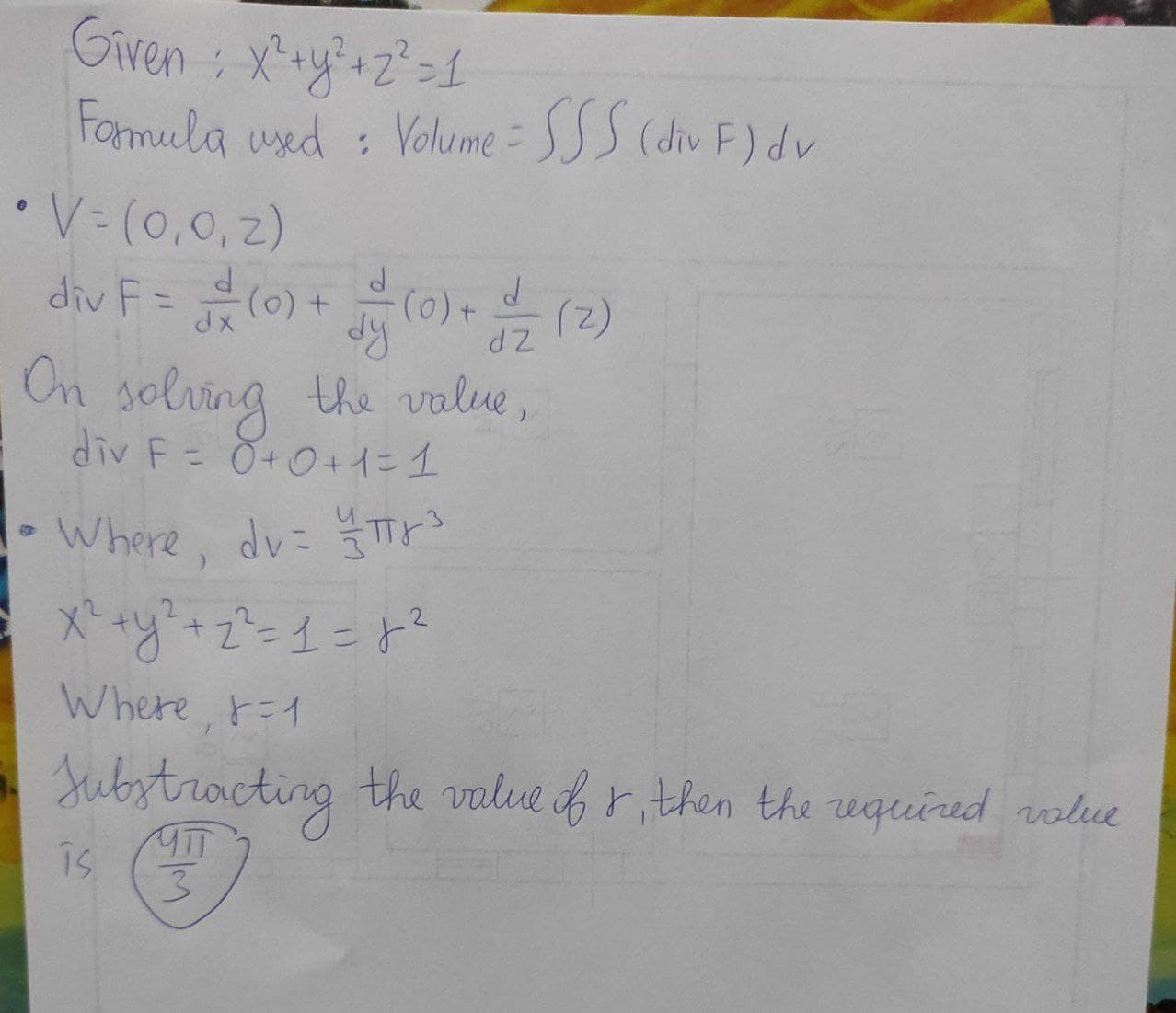# Let v = zk be the velocity field of a fluid in R^3. Calculate the flow rate through the upper hemisphere (z > 0) of the sphere x^2 + y^2 + z^2 = 1.midtlinjeg 2021-02-25 Answered

Let $v=zk$ be the velocity field of a fluid in ${R}^{3}$. Calculate the flow rate through the upper hemisphere $\left(z>0\right)$ of the sphere ${x}^{2}+{y}^{2}+{z}^{2}=1.$

You can still ask an expert for help

• Questions are typically answered in as fast as 30 minutes

Solve your problem for the price of one coffee

• Math expert for every subject
• Pay only if we can solve itSzeteib

Formula used:
Volume $=\int \int \int \left(÷F\right)dv$
$V=\left(0,0,z\right)$
$÷F=\frac{d}{dx}\left(0\right)+\frac{d}{dy}\left(0\right)+\frac{d}{dz}\left(z\right)$
On solving the value,
$÷F=0+0+1=1$
Volume $=\int \int \int \left(÷F\right)dv$
Where,
$dv=\frac{4}{3}\pi {r}^{3}$
${x}^{2}+{y}^{2}+{z}^{2}=1={r}^{2}$
Where, $r=1$
Substituting the value of r, then the required value is $4\pi /3$